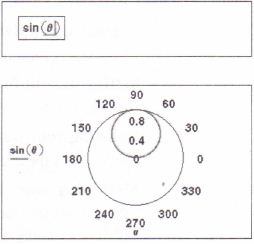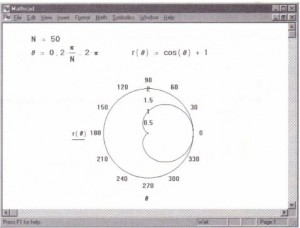Creating a polar plot

Mathcad’s polar plot operator lets you plot functions that do not lend themselves well to Cartesian (x- y) coordinates. A typical polar plot shows the value of a radial expression r versus an angular expression e. For example, you could use a polar plot to graph circular antenna patterns or electric field intensities around an object. Mathcad draws polar plots by mapping rand e onto x and y using the standard transformations x = rcos(e) and y = rsin(e) . rand e can assume positive or negative values.

You insert the polar plot operator into a blank space in your worksheet by choosing anyone of three equivalent methods: by choosing Graph-e-Polar Plot from the Insert menu, by typing [Ctrl]7, or by clicking the Polar Plot button in the Graph Palette. Like the X-Y plot operator described in Chapter 20, “Graphs,” the polar plot has placeholders on its axes for you to fill in that specify expressions to be computed and displayed, and it automatically chooses a default range for the angular axis variable if you do not specify one. In the case where you do not specify the angular axis variable, Mathcad creates a polar QuickPlot.

Polar QuickPlot

You can quickly and easily create a polar plot from a single Mathcad expression. To do so:

.” • Enter the expression or function of a single variable you want to plot. Make sure the editing lines remain in the expression.
• Choose Graphee Polar Plot from the 90
Insert menu. 120 60 Press [Enter] or click away from the 30 • graph.Mathcad automatically produces a polar plot over a default range of the dependent variable on the angular axis: 0 to 360 degrees (0 to 21tradians). You can graph multiple expressions on the radial axis, generating several traces on the polar plot, by entering them as described in “Graphing more than one expression” on page 496. Notice that you can create a QuickPlot in one of two ways. You can either:
• First type an expression and then choose Graphee-Polar Plot from the Insert menu, in which case your expression appears automatically in the radial axis placeholder;
• First choose Graphee Polar Plot from the Insert menu and then enter an expression in the radial axis placeholder.

Explicitly specifying the range on the angular axis

When you want to specify the angular axis argument or the range variable used, use the following steps to create a polar plot:
• Click where you want the polar plot to appear.
• Choose Graph-e.Polar Plot from the Insert menu. Mathcad shows a circle with four placeholders as shown below

• Above the plot region, define an angle 8 and a function of the angle, r(8).
• The bottom placeholder holds the angle variable to plot against. Enter a range variable or any expression involving a range variable in this placeholder.
• The left placeholder holds a radial expression to plot.
• The two placeholders to the right hold the upper and lower radial limits. Mathcad fills in these placeholders by default. If you want, you can modify these limits. See the section “Formatting the axes” on page 498. Just as with an equation or other plot types, Mathcad will not process the polar plot until you click outside the plot.
You can specify many of the characteristics of the polar plot including the size, the number of grid lines, and the upper and lower radial axis limits. The procedures for specifying these characteristics are described later in this chapter.

Here are the typical steps in plotting a function like:
• Define a range and an increment for 8. If you don’t, Mathcad generates a polar plot over a default range of 0 to 360 degrees.
• Define r(8), a function of 8.
• Show r(8) in a polar plot.Posted on November 20, 2015 in Polar Plots